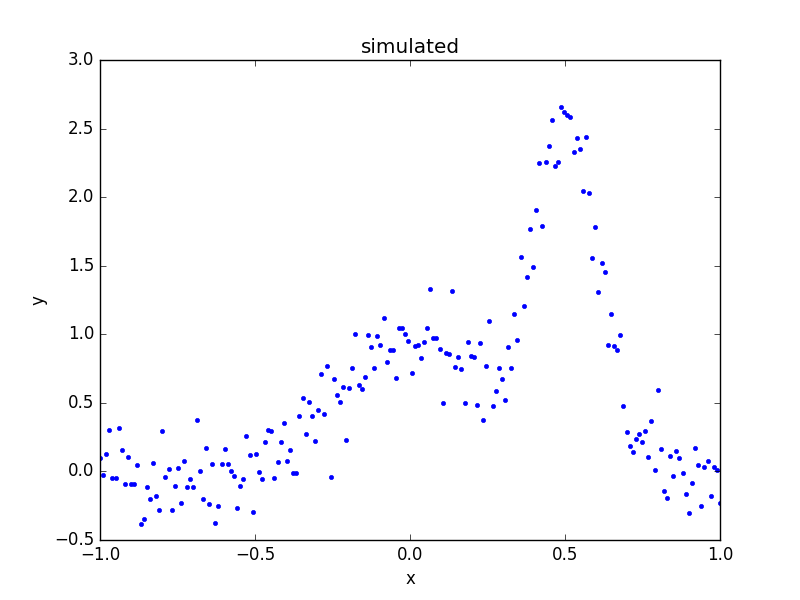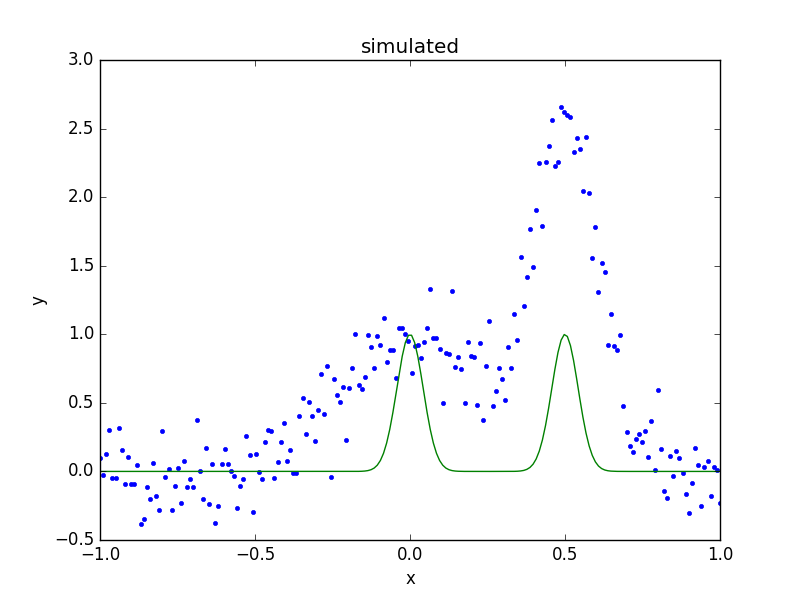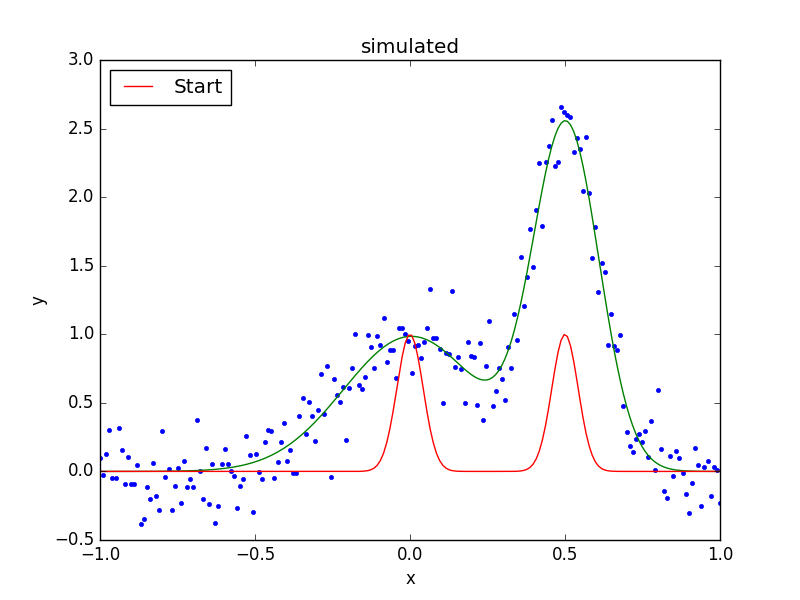Combining models and parameters¶

Most of the examples show far have used a single model component, such as a one-dimensional polynomial or a two-dimensional gaussian, but individual components can be combined together by addition, multiplication, subtraction, or even division. Components can also be combined with scalar values or - with great care - NumPy vectors. Parameter values can be “combined” by linking them together using mathematical expressions. The case of one model requiring the results of another model is discussed in the convolution section.

Note

There is currently no restriction on combining models of different types. This means that there is no exception raised when combining a one-dimensional model with a two-dimensional one. It is only when the model is evaluated that an error may be raised.

Model Expressions¶

A model, whether it is required to create a sherpa.fit.Fit object or the argument to the sherpa.ui.set_source() call, is expected to behace like an instance of the sherpa.models.model.ArithmeticModel class. Instances can be combined as numeric types since the class defines methods for addition, subtraction, multiplication, division, modulus, and exponentiation.

This means that Sherpa model instances can be combined with other Python terms, such as the weighted combination of model components cpt1, cpt2, and cpt3:

cpt1 * (cpt2 + 0.8 * cpt3)

Since the models are evaluated on a grid, it is possible to include a NumPy vector in the expression, but this is only possible in restricted situations, when the grid size is known (i.e. the model expression is not going to be used in a general setting).

Example¶

The following example fits two one-dimensional gaussians to a simulated dataset. It is based on the AstroPy modelling documentation, but has linked the positions of the two gaussians during the fit.

>>> import numpy as np
>>> import matplotlib.pyplot as plt
>>> from sherpa import data, models, stats, fit, plot

Since the example uses many different parts of the Sherpa API, the various modules are imported directly, rather than their contents, to make it easier to work out what each symbol refers to.

Note

Some Sherpa modules re-export symbols from other modules, which means that a symbol can be found in several modules. An example is sherpa.models.basic.Gauss1D, which can also be imported as sherpa.models.Gauss1D.

Creating the simulated data¶

To provide a repeatable example, the NumPy random number generator is set to a fixed value:

>>> np.random.seed(42)

The two components used to create the simulated dataset are called sim1 and sim2:

>>> s1 = models.Gauss1D('sim1')
>>> s2 = models.Gauss1D('sim2')

The individual components can be displayed, as the __str__ method of the model class creates a display which includes the model expression and then a list of the paramters:

>>> print(s1)
sim1
Param        Type          Value          Min          Max      Units
-----        ----          -----          ---          ---      -----
sim1.fwhm    thawed           10  1.17549e-38  3.40282e+38
sim1.pos     thawed            0 -3.40282e+38  3.40282e+38
sim1.ampl    thawed            1 -3.40282e+38  3.40282e+38

The pars attribute contains a tuple of all the parameters in a model instance. This can be queried to find the attributes of the parameters (each element of the tuple is a Parameter object):

>>> [p.name for p in s1.pars]
['fwhm', 'pos', 'ampl']

These components can be combined using standard mathematical operations; for example addition:

>>> sim_model = s1 + s2

The sim_model object represents the sum of two gaussians, and contains both the input models (using different names when creating model components - so here sim1 and sim2 - can make it easier to follow the logic of more-complicated model combinations):

>>> print(sim_model)
(sim1 + sim2)
Param        Type          Value          Min          Max      Units
-----        ----          -----          ---          ---      -----
sim1.fwhm    thawed           10  1.17549e-38  3.40282e+38
sim1.pos     thawed            0 -3.40282e+38  3.40282e+38
sim1.ampl    thawed            1 -3.40282e+38  3.40282e+38
sim2.fwhm    thawed           10  1.17549e-38  3.40282e+38
sim2.pos     thawed            0 -3.40282e+38  3.40282e+38
sim2.ampl    thawed            1 -3.40282e+38  3.40282e+38

The pars attribute now includes parameters from both components, and so the fullname attribute is used to discriminate between the two components:

>>> [p.fullname for p in sim_model.pars]
['sim1.fwhm', 'sim1.pos', 'sim1.ampl', 'sim2.fwhm', 'sim2.pos', 'sim2.ampl']

Since the original models are still accessible, they can be used to change the parameters of the combined model. The following sets the first component (sim1) to be centered at x = 0 and the second one at x = 0.5:

>>> s1.ampl = 1.0
>>> s1.pos = 0.0
>>> s1.fwhm = 0.5
>>> s2.ampl = 2.5
>>> s2.pos = 0.5
>>> s2.fwhm = 0.25

The model is evaluated on the grid, and “noise” added to it (using a normal distribution centered on 0 with a standard deviation of 0.2):

>>> x = np.linspace(-1, 1, 200)
>>> y = sim_model(x) + np.random.normal(0., 0.2, x.shape)

These arrays are placed into a Sherpa data object, using the Data1D class, since it will be fit below, and then a plot created to show the simulated data:

>>> d = data.Data1D('multiple', x, y)
>>> dplot = plot.DataPlot()
>>> dplot.prepare(d)
>>> dplot.plot()What is the composite model?¶

The result of the combination is a BinaryOpModel, which has op, lhs, and rhs attributes which describe the structure of the combination:

>>> sim_model
<BinaryOpModel model instance '(sim1 + sim2)'>
>>> sim_model.op
>>> sim_model.lhs
<Gauss1D model instance 'sim1'>
>>> sim_model.rhs
<Gauss1D model instance 'sim2'>

There is also a parts attribute which contains all the elements of the model (in this case the combination of the lhs and rhs attributes):

>>> sim_model.parts
(<Gauss1D model instance 'sim1'>, <Gauss1D model instance 'sim2'>)
>>> for cpt in sim_model.parts:
...:     print(cpt)
sim1
Param        Type          Value          Min          Max      Units
-----        ----          -----          ---          ---      -----
sim1.fwhm    thawed          0.5  1.17549e-38  3.40282e+38
sim1.pos     thawed            0 -3.40282e+38  3.40282e+38
sim1.ampl    thawed            1 -3.40282e+38  3.40282e+38
sim2
Param        Type          Value          Min          Max      Units
-----        ----          -----          ---          ---      -----
sim2.fwhm    thawed         0.25  1.17549e-38  3.40282e+38
sim2.pos     thawed          0.5 -3.40282e+38  3.40282e+38
sim2.ampl    thawed          2.5 -3.40282e+38  3.40282e+38

As the BinaryOpModel class is a subclass of the ArithmeticModel class, the combined model can be treated as a single model instance; for instance it can be evaluated on a grid by passing in an array of values:

>>> sim_model([-1.0, 0, 1])
array([  1.52587891e-05,   1.00003815e+00,   5.34057617e-05])

Setting up the model¶

Rather than use the model components used to simulate the data, new instances are created and combined to create the model:

>>> g1 = models.Gauss1D('g1')
>>> g2 = models.Gauss1D('g2')
>>> mdl = g1 + g2

In this particular fit, the separation of the two models is going to be assumed to be known, so the two pos parameters can be linked together, which means that there is one less free parameter in the fit:

>>> g2.pos = g1.pos + 0.5

The FWHM parameters are changed as the default value of 10 is not appropriate for this data (since the independent axis ranges from -1 to 1):

>>> g1.fwhm = 0.1
>>> g2.fwhm = 0.1

The display of the combined model shows that the g2.pos parameter is now linked to the g1.pos value:

>>> print(mdl)
(g1 + g2)
Param        Type          Value          Min          Max      Units
-----        ----          -----          ---          ---      -----
g1.fwhm      thawed          0.1  1.17549e-38  3.40282e+38
g1.pos       thawed            0 -3.40282e+38  3.40282e+38
g1.ampl      thawed            1 -3.40282e+38  3.40282e+38
g2.fwhm      thawed          0.1  1.17549e-38  3.40282e+38
g2.pos       linked          0.5     expr: (g1.pos + 0.5)
g2.ampl      thawed            1 -3.40282e+38  3.40282e+38

Note

It is a good idea to check the parameter ranges - that is their minimum and maximum values - to make sure they are appropriate for the data.

The model is evaluated with its initial parameter values so that it can be compared to the best-fit location later:

>>> ystart = mdl(x)

Fitting the model¶

The initial model can be added to the data plot either directly, with matplotlib commands, or using the ModelPlot class to overlay onto the DataPlot display:

>>> mplot = plot.ModelPlot()
>>> mplot.prepare(d, mdl)
>>> dplot.plot()
>>> mplot.plot(overplot=True)As can be seen, the initial values for the gaussian positions are close to optimal. This is unlikely to happen in real-world situations!

As there are no errors for the data set, the least-square statistic (LeastSq) is used (so that the fit attempts to minimise the separation between the model and data with no weighting), along with the default optimiser:

>>> f = fit.Fit(d, mdl, stats.LeastSq())
>>> res = f.fit()
>>> res.succeeded
True

When displayig the results, the FitPlot class is used since it combines both data and model plots (after updating the mplot object to include the new model parameter values):

>>> fplot = plot.FitPlot()
>>> mplot.prepare(d, mdl)
>>> fplot.prepare(dplot, mplot)
>>> fplot.plot()
>>> plt.plot(x, ystart, label='Start')
>>> plt.legend(loc=2)As can be seen below, the position of the g2 gaussian remains linked to that of g1:

>>> print(mdl)
(g1 + g2)
Param        Type          Value          Min          Max      Units
-----        ----          -----          ---          ---      -----
g1.fwhm      thawed     0.515565  1.17549e-38  3.40282e+38
g1.pos       thawed   0.00431538 -3.40282e+38  3.40282e+38
g1.ampl      thawed     0.985078 -3.40282e+38  3.40282e+38
g2.fwhm      thawed     0.250698  1.17549e-38  3.40282e+38
g2.pos       linked     0.504315     expr: (g1.pos + 0.5)
g2.ampl      thawed      2.48416 -3.40282e+38  3.40282e+38

The pars attribute of a model instance provides access to the individual Parameter objects. These can be used to query - as shown below - or change the model values:

>>> for p in mdl.pars:
...:         print("{:10s} -> {:.3f}".format(p.fullname, p.val))
...:     else:
g1.fwhm    -> 0.516
g1.pos     -> 0.004
g1.ampl    -> 0.985
g2.fwhm    -> 0.251
g2.pos     -> link to (g1.pos + 0.5)
g2.ampl    -> 2.484

The linked parameter is actually an instance of the CompositeParameter class, which allows parameters to be combined in a similar manner to models:

>>> g2.pos
<Parameter 'pos' of model 'g2'>
>>> print(g2.pos)
val         = 0.504315379302
min         = -3.40282346639e+38
max         = 3.40282346639e+38
units       =
frozen      = True
default_val = 0.504315379302
default_min = -3.40282346639e+38
default_max = 3.40282346639e+38